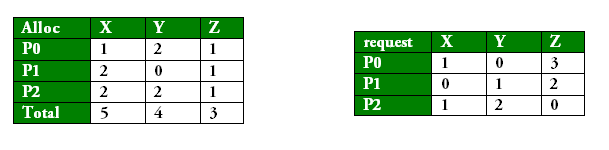Skip to content
Related Articles
GATE | GATE-CS-2007 | Question 57
• Last Updated : 14 Feb, 2018

A single processor system has three resource types X, Y and Z, which are shared by three processes. There are 5 units of each resource type. Consider the following scenario, where the column alloc denotes the number of units of each resource type allocated to each process, and the column request denotes the number of units of each resource type requested by a process in order to complete execution. Which of these processes will finish LAST?

```
alloc           request
X Y Z            X Y Z
P0  1 2 1            1 0 3
P1  2 0 1            0 1 2
P2  2 2 1            1 2 0 ```

(A) P0
(B) P!
(C) P2
(D) None of the above, since the system is in a deadlock

Answer: (C)

Explanation: A single processor system has three resource types X, Y and Z, which are shared by three processes. There are 5 units of each resource type.

So, the resource instances which are left being unallocated = <0,1,2> { unallocated resources= total resources-allocated resources }now, from the request table, you can say that only request of P1 can be satisfied. So P1 can finish its execution first. Once P1 is done, it releases 2, 0 and 1 units of X, Y and Z
respectively which were allocated to P1.So, Now unallocated resource instance are = <0,1,2> +<2,0,1> = <2,1,3>

Now Among P0 and P2, needs of P0 can only be satisfied. So P0 finishes its execution. Once P2 is done, It releases 2,2,and 1 units of X,Y and Z respectively which were allocated to P2.SO, Now unallocated resource instance are= <2,1,3> + <2,2,1> = <4,3,4>. Finally, P2 finishes its execution. So, P2 is the process which finishes in end.

Option (C) is the correct answer.

See question 2 of http://www.geeksforgeeks.org/operating-systems-set-13/

This solution is contributed by Nitika Bansal

Quiz of this Question

Attention reader! Don’t stop learning now. Learn all GATE CS concepts with Free Live Classes on our youtube channel.

My Personal Notes arrow_drop_up